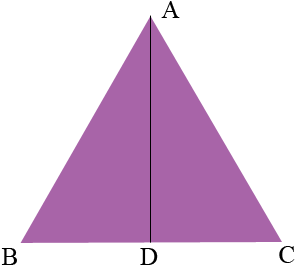SEARCH HOMEMath Central Quandaries & QueriesQuestion from Carley, a student: Hi my name is Carley. I am an 8th grader. What us the height of an equilateral triangle if the sides are 18 cm?Hi Carley,

Draw a diagram.D is the midpoint of BC and hence |CA| = 18 cm and |DC| = 9 cm. Angle ADC is a right angle. Do you see why? What does Pythagoras' theorem tell you about |AD|?

PennyMath Central is supported by the University of Regina and The Pacific Institute for the Mathematical Sciences.